## 使用MATLAB进行数字信号处理-第2部分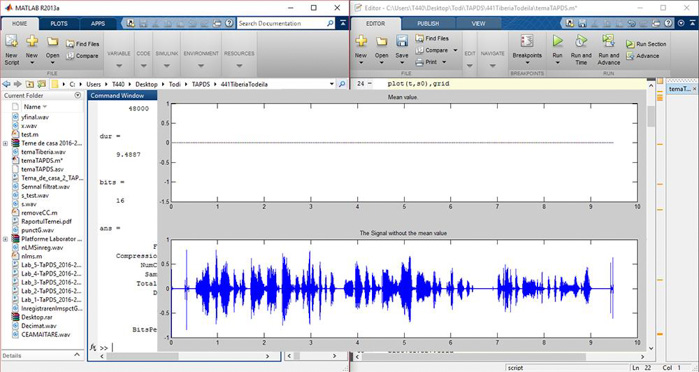• Matlab

## 第1步：如何将信号导入 Matlab

• Y – 信号名称
• Fs – 信号的采样率
• Bits – 位数

``````[s0,fs,bits] = wavread('testSound');
sound(s0,fs);
pause(9)
t=(0:length(s0)-1)/fs;
figure
plot(t,s0),grid
title('The initial signal.');
xlabel ('tTime')
ylabel('s0(n)');
``````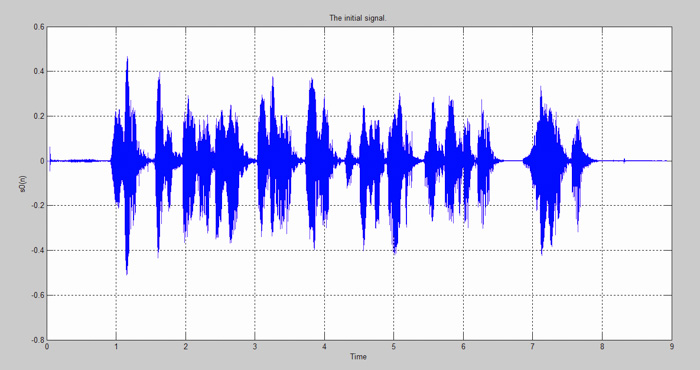``````audioinfo('testSound.wav')
``````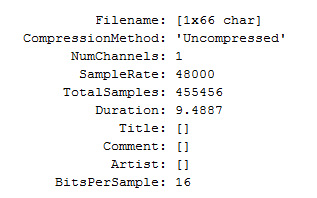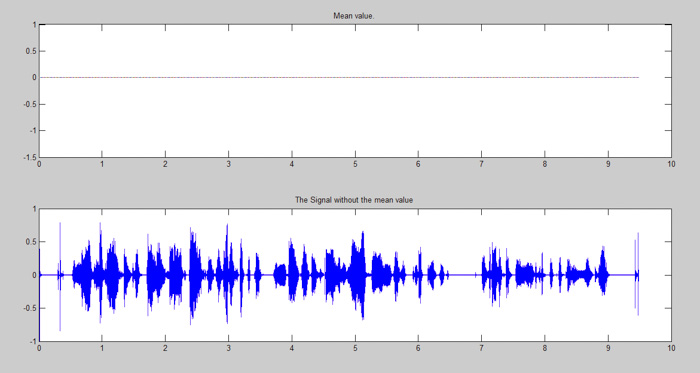## 第2步：去除平均值（或DC分量）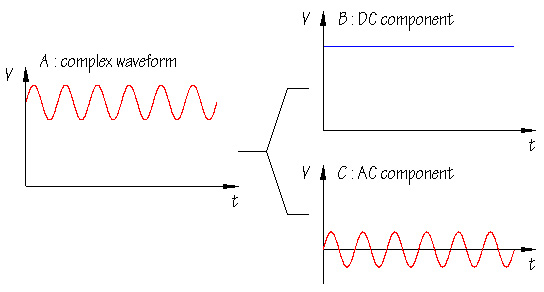``````M = mean(s0)
figure
subplot(2,1,1), plot(t,M)
title('Mean value.');
s1 = removeDC(s0);
subplot(2,1,2), plot(t,s1)
title('The Signal without the mean value');
``````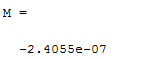``````function [sOut] = removeDC(sInput)
DC = mean(sInput);
sOut = sInput - DC;
end
``````

## 第3步：抽取过程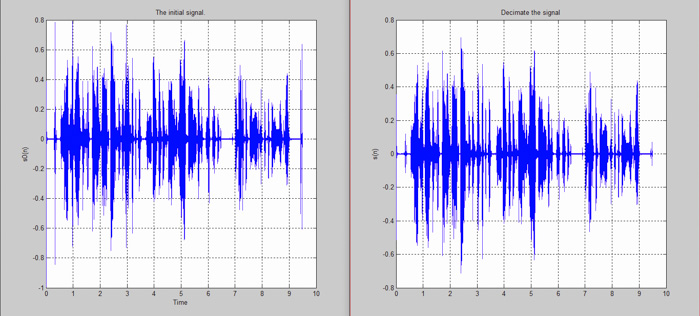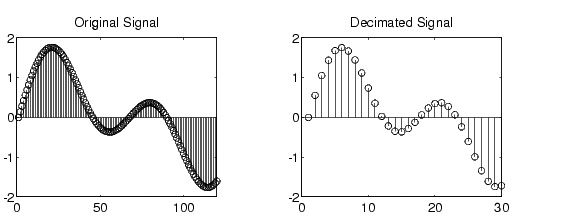``````fe2=fs/3;
%s2 = decimate(s1,3);

s2 = decimate(s0,3);
t1=linspace(0,length(s2)/fe2, length(s2));
figure
plot(t1,s2),grid
title('Decimate the signal');
ylabel('s(n)');
sound(s2,fe2);
pause(9)
``````

## 第4步：信号滤波

• 通带：一个滤波器允许通过的频率带。
• 截止频率：用户选择的某个频率，表示可以通过滤波器的理论频率。为了避免走样，此频率必须低于 采样频率/2 。在电子学中，截止频率是指信号功率变小两倍的频率值。
• 阻带：所有高于此频段的频率都无法通过滤波器。

FIR/IIR滤波器最重要的特性之一是相位特性：

• FIR: 线性相位特性
• IIR:非线性相位特性（或相位失真）

FIR滤波器属性 – dspGuru by Iowegian International

“线性相位是指滤波器的相位响应是频率的线性（直线）函数（不包括+/-180度的相位缠绕）。这使得通过滤波器的所有频率的延迟都相同。因此，这种滤波器不会引起“相位失真”或“延迟失真”。在某些系统中，比如在数字数据调制解调器中，没有相位/延迟失是FIR滤波器相对于IIR和模拟滤波器的关键优势。”

MATLAB中有众多类型的数字滤波器可供选择，但是我们需要了解如何将其应用于我们的具体场景。对于本项目，我们选用IIR滤波器，因为它更有效，即与FIR滤波器相比，我们需要更小的阶数。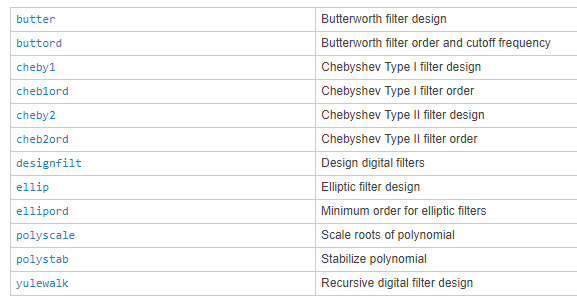``````fn = fe2/2;
fc=3400; % cutoff frequency
``````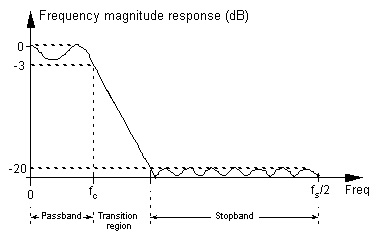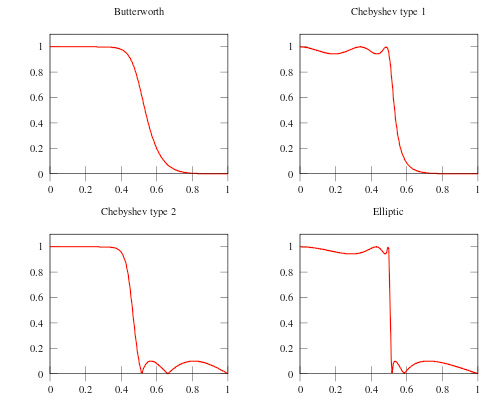IIR滤波器的阶数小于FIR滤波器的阶数。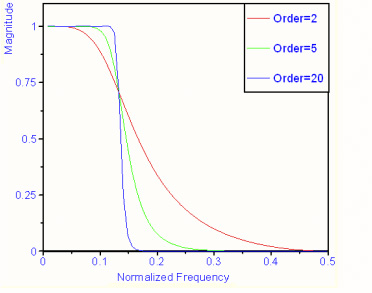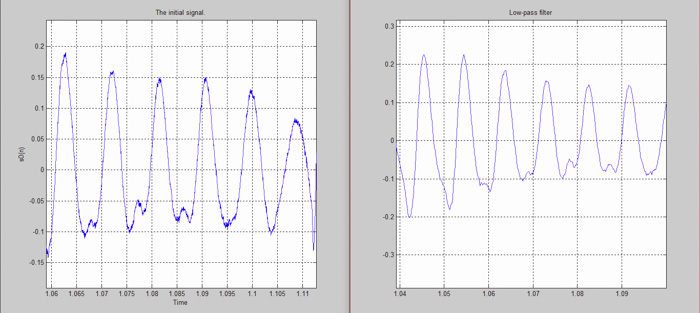##### Tiberia Todeila

Tiberia目前是布加勒斯特理工大学电气工程学院的大四学生。她非常热衷于智能家居设备的设计和开发，旨在让我们的日常生活更加轻松。

### 相关文章

1.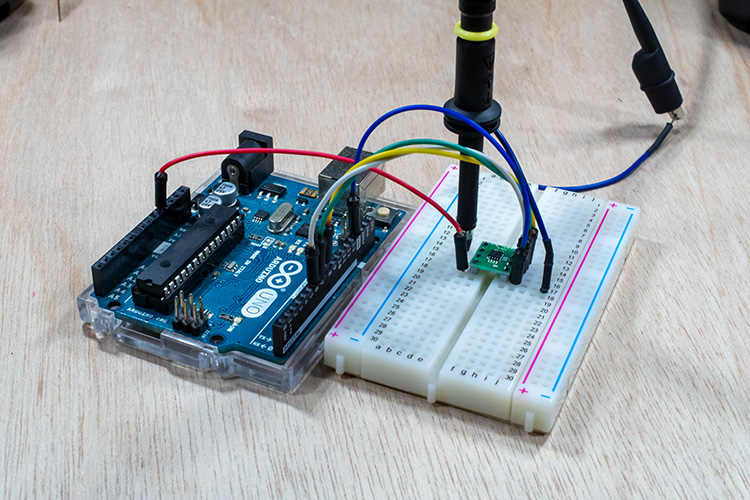#### 使用D/A转换器灵活控制电压并使用Arduino输出模拟信号的方法

2.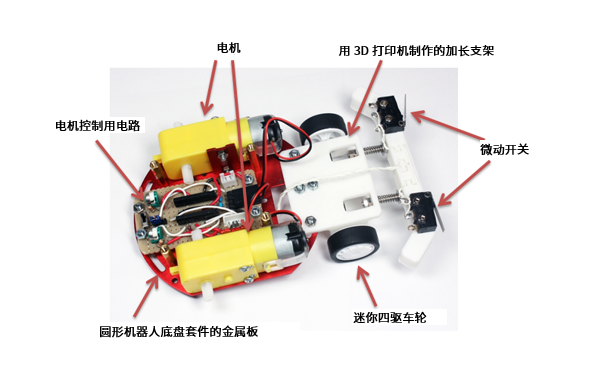#### 在最后一刻停下来！用Arduino和距离传感器制作小鸡赛车！（第3篇•最终篇）

3.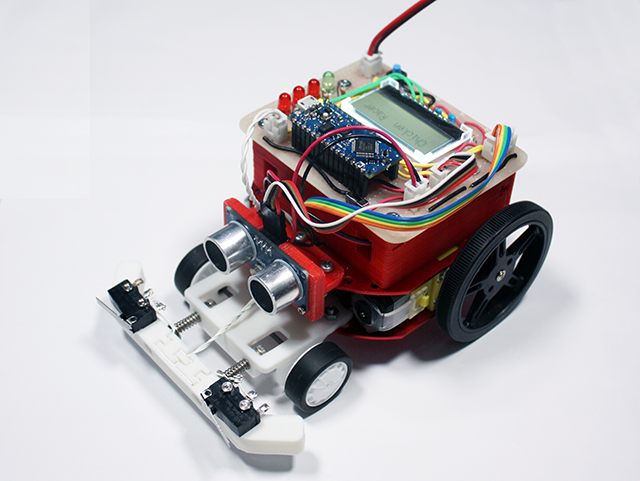#### 在最后一刻停下来！用Arduino和距离传感器制作小鸡赛车！（第2篇）

4.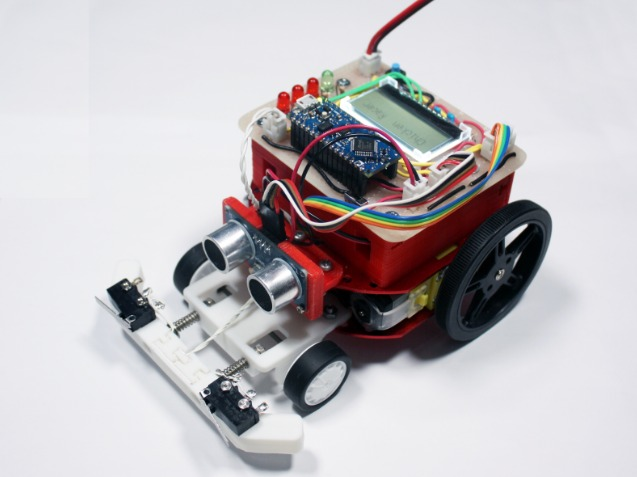#### 在最后一刻停下来！用Arduino和距离传感器制作小鸡赛车！（第1篇）

5.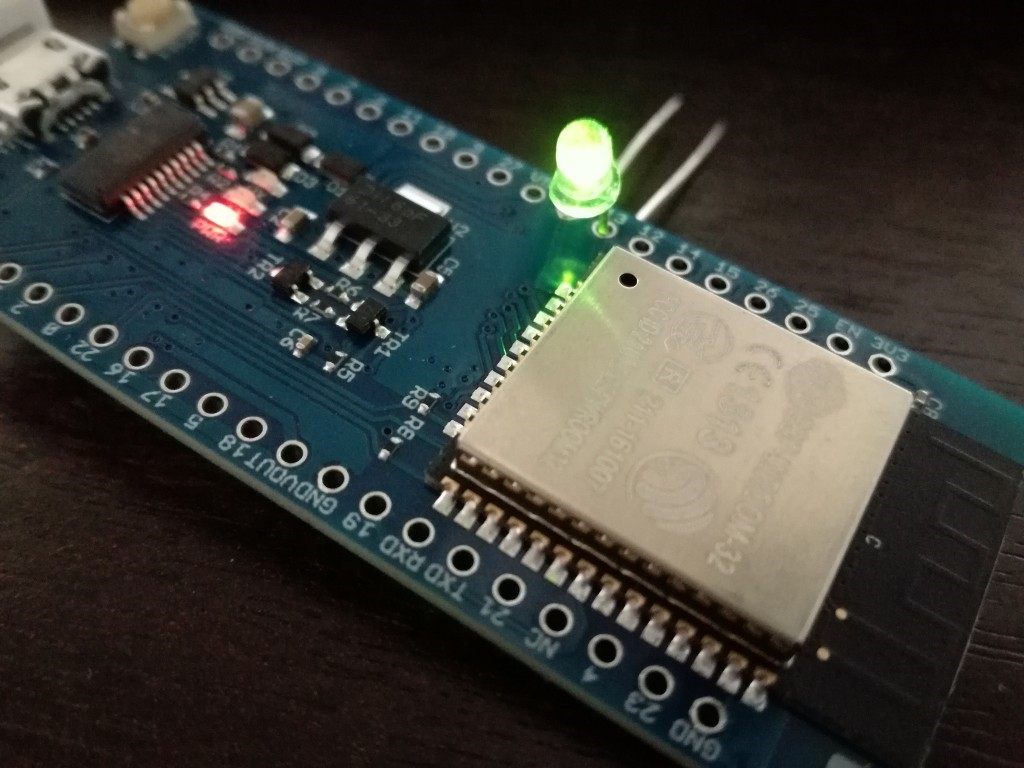#### 通过Arduino使用ESP-WROOM-32（环境搭建和配置-LED闪烁篇）

6.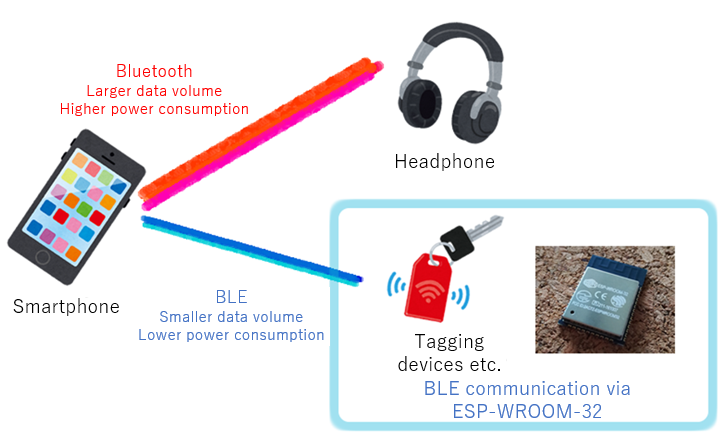#### 通过Arduino使用ESP-WROOM-32（Bluetooth-BLE连接篇）

7.#### 六种可以用于扩展物联网应用的DIY项目

8.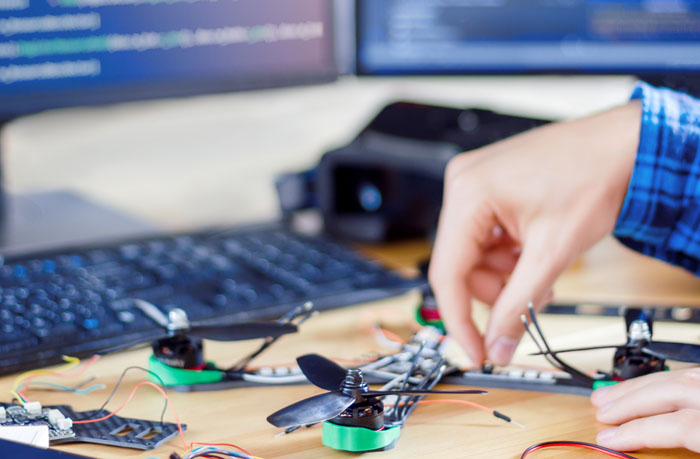#### 使用Arduino的五大DIY无人机项目

9.